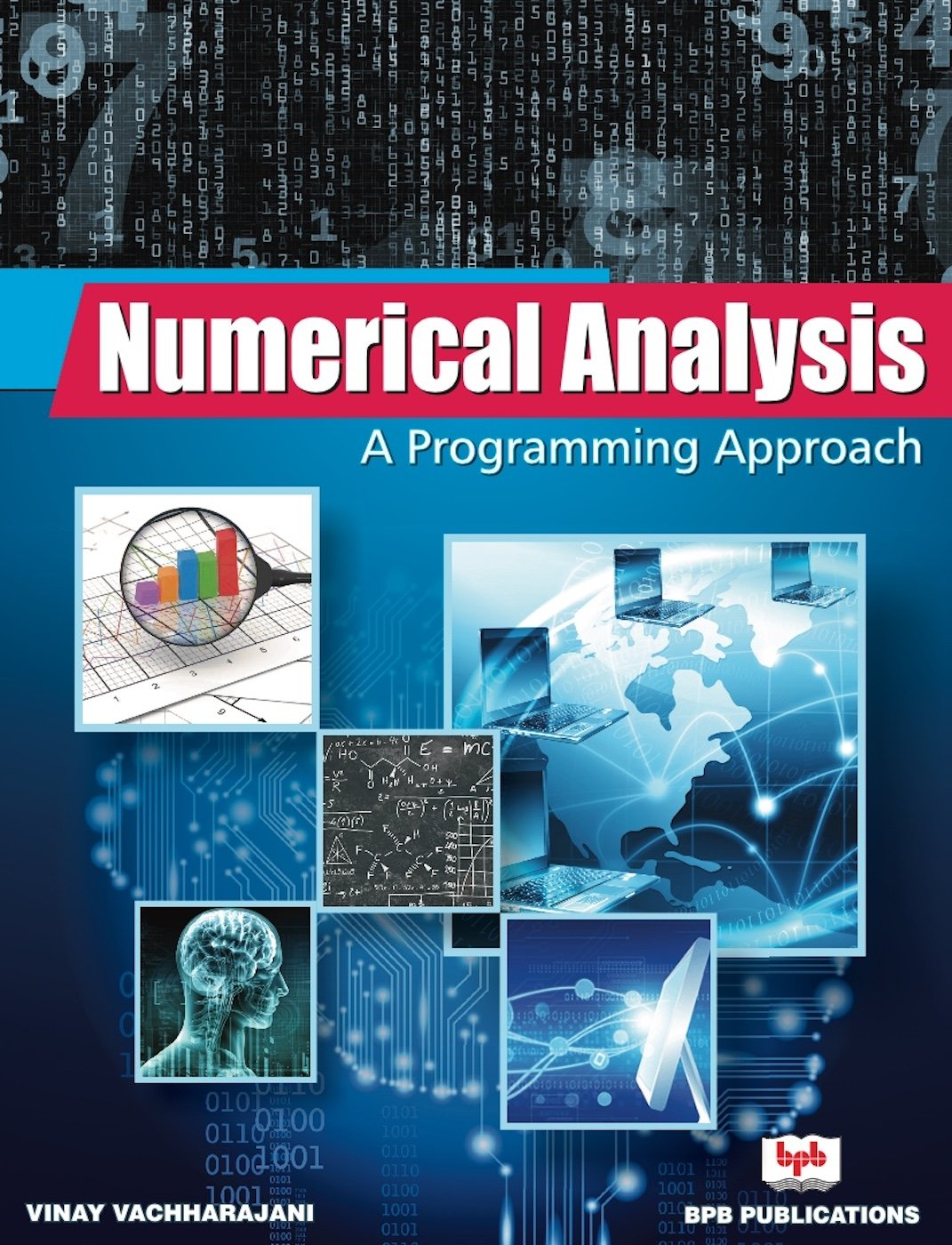# Numerical Analysis: A Programming Approach# Numerical Analysis: A Programming Approach

• Length: 596 pages
• Edition: 1
• Language: English
• Publisher: BPB Publications
• Publication Date: 2018-06-18

Learn the concept of algorithms from beginners to advanced level

Key Features

• Chapters include objectives, learning outcomes, multiple choice questions, exercises for practice and solutions.
• Programs are written in C Language for Numerical methods.
• Topics are explained with suitable examples.
• Arrangement (Logical order), clarity, detailed presentation and explanation of each topic with numerous solved and unsolved examples.
• Concise but lucid and student friendly presentation for derivation of formulas used in various numerical methods.

Description
In the present scenario where the knowledge of computers has become an absolute necessity, there has been an increasing desire for numerical answers to any applied problem. This has led to high demand for courses in numerical analysis. Many real world problems are solved first by converting them into mathematical models and then applying analytical methods to solve that model. In several cases, where ordinary analytical methods fail, numerical methods can give the result.
The book is divided into twelve chapters, describing the concept of computer arithmetic, errors, iterative methods to find the roots of transcendental and algebraic equations, curve fitting, numerical differentiation, integration, and so on.
In the book the major emphasis is given on numerous solved and unsolved examples. Many topics in this book such as process graph, cubic spline method, Taylor series, Legendre and Chebyshev polynomials, Gauss – Chebyshev integration method and boundary value problems make this book unique.

#### Tận hưởng giấc ngủ ngon với Nghe truyện thiền Khúc sông thanh bình